# The development of a full range analytical interatomic potential†

X. W. Shenga and K. T. Tang*b
aDepartment of Physics, Anhui Normal University, Anhui, Wuhu 24100, China
bDepartment of Physics, Pacific Lutheran University, Tacoma, Washington 98447, USA. E-mail: tangka@plu.edu

Received 2nd August 2020 , Accepted 8th September 2020

First published on 9th September 2020

A chronological account is given to the development of a full range interatomic potential. Starting with a simple phenomenological model, the terms in the model are gradually modified, so that they can carry some definite physical meaning. To gain insight, a systematic, order by order interaction potential theory is developed. Conversely, this theory suggests the functional form for the potential model. At present, we have a simple interaction model that is capable of describing the van der Waals potentials of many systems from R = 0 to R → ∞.

## I Introduction

The importance of the interatomic potential is succinctly put by Feynman in his ubiquitous lectures:1

If, in some cataclysm, all of scientific knowledge were to be destroyed, and only one sentence passed on to the next generation of creatures, what statement would contain the most information in the fewest words? I believe it is the atomic hypothesis that all things are made of atoms, attracting each other when they are a little distance apart, but repelling upon being squeezed into each other.

In the second part of that one sentence, he is talking about interatomic potential.

Almost all physical phenomena, outside the atomic nucleus, can be attributed directly or indirectly to the forces between atoms. Basic concepts such as temperature, pressure, viscosity of liquid and strength of solid are all intimately related to the interatomic potentials.

With modern quantum chemical methods and the advent of powerful computers, the interatomic potentials can in principle be calculated. Indeed for some rare gas systems, such as He2 and Ar2, very accurate ab initio calculations have been carried out.2–6 Such calculations are very important in that they provide the benchmark references. However, such calculations are very complicated and expensive and potentials are obtained in numerical form only. Furthermore, in the highly repulsive region, quantum chemical calculations still have difficulties.7 Therefore there is a long standing interest in simple yet reliable analytic models of interaction potentials, which should ideally consist of physically identifiable terms and which should be based on a limited number of available theoretical or empirical data.

The foundation of the theory of interatomic potentials was laid in the year of 1927. In that year the chemical bond of the singlet state of H2 molecule was successfully described by Heitler and London8 using a symmetrized wave function. The calculation showed that the stabilization of the bond is provided by the exchange integral. The same calculation also demonstrated that the exchanged integral is responsible for the repulsive potential of the triplet state. In the same year Wang9 pointed out that there is always a long range attraction between any pair of atoms, which is now known as the dispersion energy, and is due to the interaction of a dipole with an instantaneous induced dipole. This long range attraction together with the exchange repulsion are the origin of the so called van der Waals potential between systems which do not form a chemical bond.

In this paper we will describe a simple model for van der Waals potentials which can accurately describe the full range potentials of many systems. We will first provide a chronological account of the development of this model and then give some examples of its applications.

Atomic units will be used throughout this paper. To facilitate comparison with experiments other units will from time to time also be used. When this is the case, it will be explicitly stated.

## II. The Toennies model

In early 1970s, the molecular beams were well developed. Spearheaded by Lee's group,10–12 the well region of interaction potential between rare gas atoms were determined to a high degree of accuracy by the scattering experiments. The combined analysis of beam scattering data, virial and transport coefficient measurements as well as liquid and solid state properties had made it possible to determine the potential curves of rare gas–rare gas interactions in the entire van der Waals region.13 It turned out that simple models, such as Lennard-Jones potential, were not adequate to describe these potential curves, and multi-parameter, piece-wise spline fits had to be used.

In 1973, Toennies published a paper14 in which he pointed out that the Buckingham potential model modified by including the R−8 and R−10 dispersion terms

 (1)
could explain the van der Waals potentials of He, Ne, Ar and Kr dimers. In this expression, the Born–Mayer parameters A and b of the repulsive potential [Aexp(−bR)] could be obtained from the recently calculated SCF values and the dispersion coefficients C6, C8 and C10 could also be estimated at the time.

This observation is the stimulus and the starting point of our journey of searching for physically transparent models of interatomic potentials.

It became clear, upon close examination of eqn (1) that the good agreement with experiment must be due to some fortuitous cancellation of errors.14 In particular, the C10 term in the equation was found to have a strong influence on well depth, contributing between 25–75% with the larger value applying to the heavier systems. While it is well known that the long range attractive potential can be described by the dispersion series obtained from the second order perturbation theory,15,16 but this is an asymptotic series and has more than three terms.17 This raises the question as to what effect the rest of the series will have. Unfortunately coefficients higher than C10 were not available at the time. Furthermore, the divergent nature of the asymptotic series was not accounted for. In other words, the dispersion series must be “damped” as R decreases.

Our first attempts to answer these questions were to use the fact that the dispersion series are asymptotic series. It is well known that the best estimate of an asymptotic series at any given R is obtained by terminating the series at the term before the smallest term and including a fraction of the smallest term.17 Thus, with increasing R the number of terms retained in the series should be increased. Based on this property, we were also able to derive a recurrence relation:18

 (2)

With the knowledge of the first three dispersion coefficients, higher ones can be estimated within a few percent. Although much more complicated recurrence relations were subsequently derived,19 tests against the accurate coefficients of He–He interaction, which became available later,20 show that eqn (2) works just as well.

With these corrections, the Toennies' model was able to predict the potentials of a large number of atom–atom,21 atom–molecule22–24 interactions.

It should be mentioned that the well known HFD (Hartree–Fock Dispersion) potential was also based on the Toennies model, except the damping of the dispersion series is modeled after the H2 interaction.25,26 There are a great many different HFD potentials in literature. Either the repulsive functional form fitting the SCF data is somewhat different, or the attractive series is changed through twiddling the dispersion coefficients and/or the empirical damping function. For example, at least six different HFD potentials, from HFD-I to HFD-D, are mentioned in ref. 27 alone. The purpose of these efforts to modify the HFD potential is to fit all experimental results into the same potential. The results of these attempts are summarized in the Aziz's report.28

## III. The TT potential model

In spite of considerable success, the Toennies potential model suffers from the fact that, after corrections, is only piece-wise continuous.

The dispersion coefficients C2n were derived by assuming that there is no overlap of the electron clouds of the two interacting atoms. This of course is not the case. We invoked the semiclassical Drude model,29 similar to the one used earlier by Kim and Gordon,30 to estimate the effect of the overlap of the electron clouds on the dispersion coefficients. It turned out that this effect can be written in such a way that the dispersion terms C2n/R2n are reduced to f2n(bR)C2n/R2n, with f2n(bR) given by

 f2n(bR) = 1 − P2n(bR)exp(−bR)
where P2n(bR) is a polynomial of order 2n and b is the same distance scaling factor as in the Born–Mayer repulsion Aexp(bR). With the following realistic boundary conditions of f2n(bR):
 f2n(bR) → 1, R → ∞
and
 f2n(bR) → 0 + 0(R2n+1), R → 0,
we obtained an universal damping function31
 (3)
which is an incomplete gamma function of order 2n + 1.

The most discriminating test was the accurate ab initio calculations of Koide, Meath and Allnatt for H(1S)–H(1S).32 They presented the damping function for 2n = 6…(2)…20. The agreement with eqn (3) in all cases is remarkably good. The agreement is best for the most important terms with 2n = 6, 8 and 10. For the higher order terms, at internuclear distances where f2n = 0.5, eqn (3) are larger by 2% for 2n = 12, and in the worst case by 7% for 2n = 20. Later, when accurate ab initio damping functions for some other systems became available,33,34 they are found to be all in good agreement with eqn (3).

Thus, with the Born–Mayer repulsion, we have the model potential31

 (4)
which is often referred as TT potential. In practice, the dispersion series has to terminate somewhere, Nmax is the largest n value.

Compare to the accurate potentials available for systems such as Ar2, the well depth De (the potential minimum) obtained from the TT potential of eqn (4) with the repulsive term Aexp(−bR) fitted to SCF ab initio data, are about 15% too deep. This is consistent with the observation from the ab initio perturbation theory that the inclusion of the correlation effects increases the magnitude of repulsion. Since this and other small effects are difficult to determine and the well depth De and its location Re are available for many systems, we propose to determine A and b from De and Re. This is most conveniently done with the reduced potential. If we define x = R/Re, then eqn (4) can be written as V(R) = DeU(x) where U(x) is the reduced potential and is defined as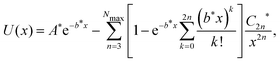with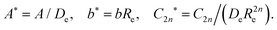At the potential minimum x = 1, the reduced potential provides us with two equations:
 U(1) = −1,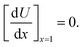These two equations are sufficient for us to find A and b. Now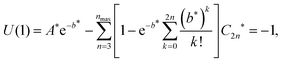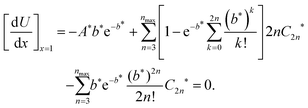Solving for A* from the second equation and substituting it into the first equation, we have

 (5)

This equation can be solved for the only unknown b*. This can be most expediently done on a computer. In the appendix of ref. 35, there is a simple program to solve this equation for the particular case of He2 with Nmax = 8. With b* we can easily calculate A*, so A = A*De and b = b*Re.

In the original paper,31 we showed that with the parameters in eqn (4) so determined, it was possible to predict the interaction potentials for not only the rare gases, but also H2(3Σ), NaK(3Σ), and LiHg complexes. Since then, this model has been successfully applied to many more systems.36–42

## IV. The TTY potential

To gain a better understanding of the interaction potential, we used the theory of the permutation group and the Rayleigh–Schrödinger perturbation theory to develop a method for systematic calculation of interatomic potentials.43,44 When this method is applied to the H2 molecule, the triplet (t) and singlet (s) energies are given by
 (6)
where εn is the nth order polarization energy (unsymatrized perturbation energy) and sn is the nth order overlap respectively, and εx is the exchange energy defined as (EsEt)/2. With M = 1, this expression is shown to be identical to the usual Heitler–London energy, therefore this method is called generalized Heitler–London (GHL) theory.45 When εx is expanded in terms of Coulomb and exchange integrals, many previous symmetry adapted perturbation theories are shown to be subsets of this expansion. The advantage of the GHL theory is that, instead of using approximate exchange integrals, the exchange energy calculated from the surface integral method can be used directly. It can be shown that46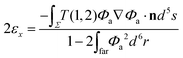where Φa is a localized wave function in the six dimensional space of two electrons, and Σ is the five dimensional surface separating the six dimensional space, and T(12) is the operator interchanging electron 1 and electron 2, and n is the unit vector perpendicular to Σ directing from near side to far side. Using only the leading term of the asymptotic radial wave function,47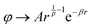where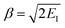with EI = the ionization energy of the atom, these integrals can be evaluated for two identical atoms. The energy of the exchange of a single pair electrons in the asymptotic region is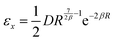with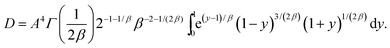In this expression an error of a factor 4.5 in the result reported by Duman and Smirnov48 has been corrected. The one dimensional integral can be evaluated and D/A4 can be fitted to the form49

 D/A4 = 0.0129 + 0.1297β − 0.0403β2
with a maximum deviation of only 0.25% for the ionization energies of all known atom. The only case we know A exactly is the hydrogen atom for which the asymptotic wave function is the exact wave function. For this case the ionization energy of the ground state is 0.5, therefore β = 1. The normalized ground state wave function is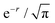which can be written as the product of radial part and angular part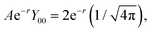so A = 2. Therefore D = 24(0.1023) = 1.6368. Thus
 εx = 0.818R2.5e−2R.

This is the asymptotic exchange energy of H2 by Herring and Flicker.50 They were the first to obtain the correct asymptotic energy. Although a number of approximations were used by Herring51,52 to obtain the effect of electron–electron correlation, he was able to show that this is the asymptotic exact result. It is interesting to note that when Herring undertook this study, the purpose was to correct the conceptual defect of the Heitler–London method. Even Herring himself thought this approach is only “good for the soul”. Later it turned out that this is in excellent agreement with the most elaborate ab initio calculation53 not only in the van der Waals region but all the way down to R ≈ 2 a.u.

The surface integral method is based on the physical process of electrons hopping between nuclei. Although this interpretation is readily accepted in the charge exchange scattering experiments, it apparently contradicts textbook wisdom. While the physical interpretation might still be controversial, the mathematical formulation is indisputable.

The theory was further extended by Smirnov and Chibisov54 to treat general atomic systems. It is based on the fundamental approximation that the exchange interaction between two multielectron atoms is dominated by the exchange of a single pair of electrons at any one time. However, in order to antisymmetrize the total wavefunction, the coupling of orbital and spin angular momenta of all equivalent electrons has to be taken into account. As a result, the exchange energy of a multielectron system is found to equal to the exchange energy of single pair multiplied by a rather complicated coupling constant k which was first calculated by Duman and Smirnov.48 Unfortunately their final results were incorrect. The correct result is given in ref. 55. Although these k factors are the result of a rather complicated angular momentum coupling, all of them are identical to the number of possible exchanges between the valence electrons with the same spin in the two atoms. For example, the k values for H–He, He–He, He–Ne and Ar–Ar interactions are respectively 1, 2, 6 and 18.

According to the GHL, the van der Waals potential is given by V = Etε0 and for M = 2,

 V = ε1 + ε2 + (1 +s0 + s1)εx

To use this theory to study the potential curves of He2, Ne2 and Ar2,56 various terms were evaluated with CI wave functions. It turn out that ε2 is very well approximated by the damped dispersion series, and ε1 is almost parallel to εx and is only about 10% of εx in magnitude. The overlaps s0 and s1 are negligibly small in the van der Waals region. Thus by decrease A in the εx by about 2.5%, the ε1 contribution can be accounted for because exchange energy depends on A4. With this modification, the van der Waals potential can be written as V = εx + ε2.

In a parameter free study of the van der Waals potential of He2,57 we used the expression of the exchange energy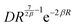for repulsion and for attraction we used the damped dispersion series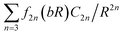with b given by the logarithmic derivative of the repulsion. Since the ionization energy of He is 0.9036, so β = 1.3443.The asymptotic amplitude A calculated from SCF is determined to be 2.84. Therefore D = 7.449. With these parameters and the known dispersion coefficients, we found a potential curve that is in amazing agreement with the no-appproximation bruteforce Monte Carlo calculations.58 This agreement is fortuitous. The CI wave function determined A is equal to 2.9156 which is 2.46% larger than 2.84 determined by the SCF wave function. Therefore the neglected first order Coulomb energy is completely compensated.

The expression for the heterogeneous molecules is far more complicated, and is currently an interesting subject for research.46,59 Furthermore, even for homonuclear dimers, the amplitude D is very difficult to determine. Therefore in our search for a simple potential model for both heterogeneous and homogeneous dimers, we used what we call the Tang–Toennies–Yiu (TTY) model60

 (7)
where the damped dispersion series is exactly the same as in the TT potential, for the repulsion the Born–Mayer potential AebR is replaced by Duman–Smirnov term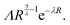It is clear that if these two expressions and their first derivative are the same at the potential minimum, then VTTY(R) will have the same well depth De at the same location Re. To satisfy these conditions, λ and Λ are required to be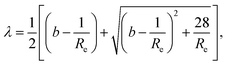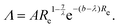Thus, the TT potential can easily be transformed into the TTY potential. We think it is an improvement because its repulsive potential has a stronger theoretical foundation. Furthermore, this functional form is softer in the intermediate distances and is in better agreement with experiment.61 The obvious disadvantage of TTY is that it turns to zero as R goes to zero.

## V. The TT2 potential

In order to extent the potential to R = 0, it is necessary to define the pure electronic part of the potential which is given by62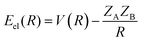where ZA and ZB are the nuclear charges of the separate atoms. Since Eel(0) is the difference between the energy of the united atom Eua and that of the two separate atoms EA and EB, and these energies are known, so Eel(0) is just a known constant
 Eel(0) = Eua − EA − EB = C.

The electronic energy must also satisfy the condition62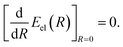Following Buckingham,63 we write the short range potential as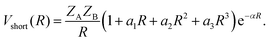The final expression for the extended potential (TT2) is given by joining the short range to the TTY potential,60

 VTT2(R) = Vshort(R) + (1 − e−αR)VTTY(R), (8)
where the term (1 − eαR) serves to gradually turn out the VTTY potential, so that it is fully replaced by Vshort at short distances.

In addition to ZA and ZB (which are known), there are four additional parameters a1, a2, a3 and α which can be determined in the following way. By expanding eαR in terms of power series, we see that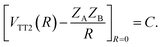(9)

Similarly, from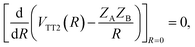we have
 (10)

Furthermore, we require that the new potential VTT2(R) is consistent with the potential parameters De and Re at the van der Waals minimum. That is

 VTT2(Re) = De,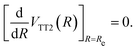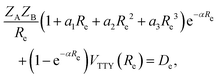which after substituting VTTY(Re) = De is reduced to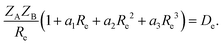ZAZB(a1 + 2a2Re + 3a3Re2) = De.

Combining the last two equations, we have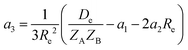and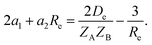Putting in a1 and a2 of eqn (9) and (10), we have a quadratic equation of α which can be solved to give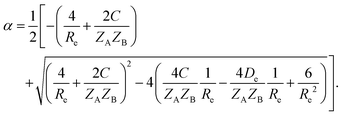Thus all parameters are determined.

For H–H, H–He, and He–He interactions, the input data and the calculated parameters of eqn (8) are shown in Table 1. This table is similar to the Table 1 in ref. 60, except in the present case we include 3 more dispersion terms. Because of that, some of the calculated parameters are different. This will facilitate our discussion of the upper limit Nmax of the dispersion series.

Table 1 Potential parameters for TT,TTY and TT2 models. All of the input parameters except for C12, C14 and C16 are taken from ref. 60. C12, C14 and C16 are calculated based on the eqn (2)
H–H H–He He–He
Input parameters
C6 6.49903 2.82134 1.46212
C8 124.399 41.8364 14.1258
C10 3285.83 871.540 183.781
C12 1.1977 × 105 2.5507 × 104 3.2199 × 103
C14 6.0239 × 106 1.0487 × 106 7.5971 × 104
C16 4.1811 × 108 6.0575 × 107 2.4138 × 106
Re 7.83 6.66 5.608
De −2.048 × 10−5 −2.256 × 10−5 −3.482 × 10−5
ZA 1 1 2
ZB 1 2 2
EA −0.49973 −0.49973 −2.90339
EB −0.49973 −2.90339 −2.90339
Eua −2.17503 −7.47798 −14.66844

Derived parameters
TT parameters
A 10.51789 5.80043 25.18501
b 1.68113 1.85898 2.41630

TTY parameters
Λ 0.74737 0.80930 7.94920
λ 2.00034 2.18899 2.70024

TT2 parameters
α1 0.90618 1.72603 1.84438
α2 −0.28040 −0.58597 −0.75316
α3 0.01895 0.04568 0.06999
α 2.08175 3.76346 4.05980

## VI. The TTS potential

Recently we found that it is useful to write the TT2 potential of eqn (8) in the following form:64
 (11)
where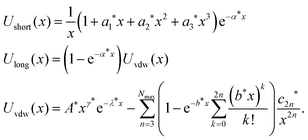with the numerical values listed in Table 1, the star quantities of this equation can be calculated. They are listed in Table 2.
Table 2 Star quantities in TTS model for H–H, H–He and He–He
Star quantities Definition H–H H–He He–He
a1* a1Re 7.09539 11.49538 10.34329
a2* a2Re2 −17.19110 −25.99090 −23.68667
a3* a3Re3 9.09555 13.49545 12.34334
α* αRe 16.30010 25.06466 22.76733
λ* λRe 15.66269 14.57866 15.14296
γ*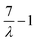2.49940 2.19783 1.59236
b* bRe 13.16329 12.38083 13.55060
A*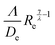6.2528 × 106 2.31539 × 106 3.5552 × 106
C6*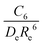1.37704 1.43308 1.34992
C8*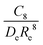0.42993 0.47909 0.41469
C10*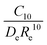0.18522 0.22501 0.17155
C12*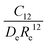0.11012 0.14847 0.09557
C14*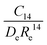0.09034 0.13762 0.07170
C16*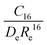0.10228 0.17921 0.07244

In this potential, both Ushort(x) and Ulong(x) are dimensionless. Other than the ZA and ZB which are the well known number of nuclear charges, the potential depends only on the well depth De and its location Re which should both be in atomic units. Note that ZAZB/Re is also energy in atomic unit. We call the potential of eqn (11), the TTS potential.

## VII. Applications

### A. A universal analytical potential for the rare gas dimers over the full range of internuclear distances

In a recent communication with this title,64 the interaction potentials of all the possible 21 homogeneous and heterogeneous pairs of rare gas atoms are determined with the TTS model. They are assumed to be conformal with the He2 interaction, therefore the star quantities of He2 in Table 2 can be used in eqn (11). Then the potential of each dimer is characterized by only four parameters: Re, De, ZA, ZB. The resulting potentials are in remarkable agreement with the available accurate ab initio calculations.64

We will not repeat these calculations here, except in this paper we carry three more dispersion terms, in other words, in ref. 64 Nmax = 5, and in this paper Nmax = 8. This enables us to discuss the effects of more dispersion terms.

Theoretically, the damped dispersion series should include all the terms necessary for convergence. This usually means that a large number of terms must be included in the series to get the correct overall potential. However, if the repulsion parameters (A and b in the TT potential, Λ and λ in the TTY potential) are determined from Re and De (like what we are doing here in solving eqn (5)) with only the first three leading dispersion terms, then this truncated model is usually adequate to predict an accurate potential in the van der Waals well region. This can be understood by noting that if Re and De are correctly reproduced, then the small error caused by dropping terms higher than the third term in the dispersion series must be compensated by the adjustment of the repulsive potential near the minimum of the potential. Moreover, for RRe the contribution from the neglected dispersion terms and the repulsive potential are all very small, and in this region the potential is dominated by the three leading dispersion terms. So there may be very little difference between the potential with three (Nmax = 5) dispersion terms and the potential with six (Nmax = 8) dispersion terms. On the other hand, for RRe, since the compensation may not be complete in this region, and more dispersion terms contribute more to the negative component of the potential, therefore the potential with Nmax = 8 may be softer than the potential with Nmax = 5. Normally we should not make Nmax much higher than 8, since higher dispersion coefficients are generally not available and the recurrence relation of eqn (2) is not precise. Repeated use of eqn (2) may produce unwarranted errors.

We illustrate this situation in Fig. 1 for the case of Ar2. In Fig. 1a, R < 6 a.u., this is the region where the potential raises rapidly, therefore it is plotted in logarithmic scale. First we see how the accuracy is improved in going from TT potential to TTY potential, then from TTY potential to TTS potential. Secondly, we see that Nmax = 8 curves are always smaller than the corresponding Nmax = 5 curves. In Fig. 1b, R > 6 a.u. This is where the bowl of the van der Waals potential of Ar2 is located. In this region, the potential is plotted in linear scale. It is seen that in this region there is not much difference between these potentials. Therefore we can say that in the van der Waals region, the potential with Nmax = 5 is a good enough approximation.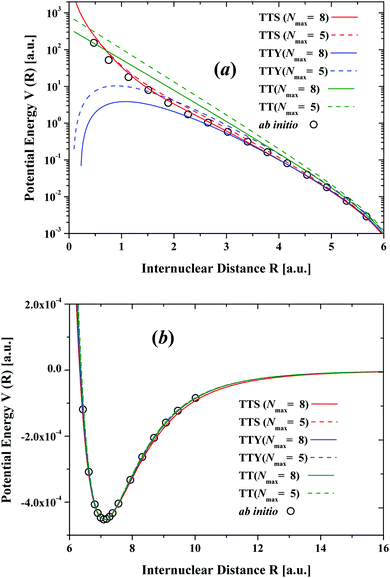Fig. 1 The interaction potential of ground state Ar2. The lines are the present result. Circles are the ab initio results taken from ref. 4 and 5.

### B. Full range analytical potentials for rare gas hydrides

It seemed reasonable to assume that the interaction potentials of H–Ne, H–Ar, H–Kr, H–Xe are conformal with that of H–He. If that is the case, then the star quantities of H–He in Table 2 can be used in eqn (11) to describe the potentials of these rare gas hydrides. The four parameters De, Re, ZA, ZB required to characterize these potentials are listed in Table 3. The nuclear charges ZA, ZB are of course well known. The De and Re are chosen from the “best experiment”.
Table 3 Potential parameters in TTS model for rare-gas hydrides
Re De × 105 ZA ZB
a Taken from ref. 65 and 67.b Taken from ref. 66 and 67.
NeH 6.425a 5.365a 10 1
ArH 6.709b 17.052b 18 1
KrH 6.898b 21.094b 36 1
XeH 7.200b 25.357b 54 1

For the H–Ne system, we chose the results of Hishinuma65 who carefully measured the absolute integral cross sections between 1.8 and 330 meV. For H–Ar, H–Kr, and H–Xe systems, the orbiting resonance experiments of Toennies et al.66 still appear to be the most sensitive. These experiments were reanalysed with more accurate dispersion coefficients and the results are shown in ref. 67. The resulting potentials are shown in Fig. 2. Comparisons with the combining rule results of Tang and Toennies67 and with the MCSCF calculations of Das et al.68 show that these TTS potentials of eqn (11) are quite accurate.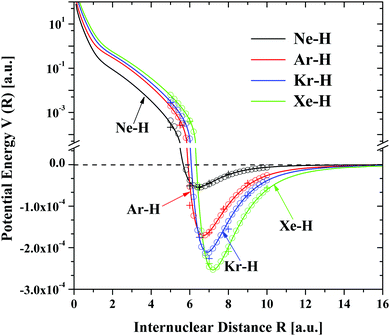Fig. 2 The interaction potential of the ground state rare-gas hydrides (Ne–H, Ar–H, Kr–H, Xe–H). The solid lines are the present results. Small circles are the combining rule results of Tang and Toennies.67 Crosses are the MCSCF results of Das et al.68

### C Full range analytical potentials for the alkali–hellium systems

The interaction potentials of Li–He, Na–He and K–He systems were studied by Partridge et al.69 using the coupled cluster single, double and perturbative triple CCSD(T) method. Here we simply use the TTS model of eqn (11) with the star quantities of H–He interaction to describe these potentials. The four required parameters De, Re, ZA, ZB are listed in Table 4. The values of De and Re are also taken directly from the paper of Partridge et al. In Fig. 3, 4 and 5, the present potentials are compared with the calculations Partridge et al. in all the data ranges. Clearly the TTS model can describe these potentials fairly accurately.
Table 4 Potential parameters in TTS model for alkali–helium
Re De × 106 ZA ZB
a
HeLi 11.47a 7.36a 2 3
HeNa 11.85a 6.96a 2 11
HeK 13.50a 5.00a 2 19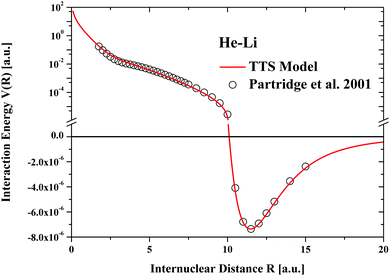Fig. 3 The interaction potential of ground state HeLi. The red line is the present result. Circles are the ab initio results taken from ref. 69.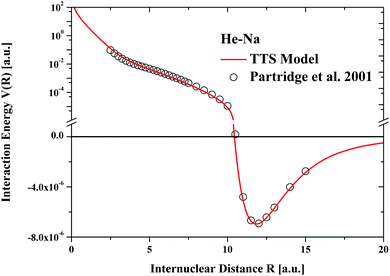Fig. 4 The interaction potential of ground state HeNa. The red line is the present result. Circles are the ab initio results taken from ref. 69.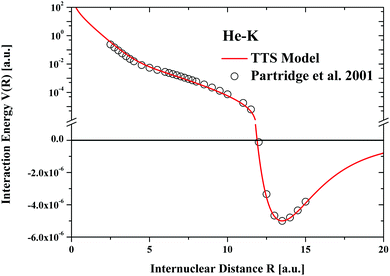Fig. 5 The interaction potential of ground state HeK. The red line is the present result. Circles are the ab initio results taken from ref. 69.

For Rb–He and Cs–He, accurate values of De and Re are not known. We could estimate them using combining rules,35,70 but they probably can be more accurately calculated in the following way. The dispersion coefficients of alkali–helium listed in the table of Jiang el al.71 are rather accurate. Since these systems conform with each other, the reduced dispersion coefficients (C6* = C6/(Re6De), C8* = C8/(Re8De) should be nearly the same for all of them. These quantities are listed in Table 5.

Table 5 Well depth De, equilibrium distance Re and dispersion coefficients for alkali–helium
Re De × 106 C6 C8 C6* C8*
a b c Calculated in the present paper.
HeLi 11.47a 7.36a 22.535b 1084.2b 1.34462c 0.49173c
HeNa 11.85a 6.96a 25.759b 1326.9b 1.33663c 0.49032c
HeK 13.50a 5.00a 39.468b 2620.7b 1.30398c 0.47509c

It is seen that the reduced dispersion coefficients C6* and C8* are indeed nearly identical for all three interactions. Now it follows from the definitions of the reduced dispersion coefficients that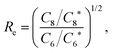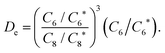Using the average values of the reduced dispersion coefficients of C6* and C8*, and the C6 and C8 values from Jiang's Table, we calculate the Re and De for all alkali–helium systems, their values are listed in Table 6. Together with ZA and ZB, The TTS potentials for these systems can be plotted.

Table 6 Dispersion coefficients and predicted Re and De for alkali–helium
C6 C8 Re De × 106 ZA ZB
HeLi 22.535 1084.2 11.47 7.45 2 2
HeNa 25.759 1326.9 11.87 6.93 2 11
HeK 39.468 2620.7 13.48 4.96 2 19
HeRb 44.785 3144.9 13.86 4.76 2 33
CsHe 52.679 4273.1 14.89 3.63 2 54

For Li–He, Na–He and K–He, they are almost indistinguishable from Fig. 3, 4, 5 respectively. Fig. 6 shows the potentials we predicted for Rb–He and Cs–He. The predicted Re and De for Rb–He and Cs–He are compared with other determinations in Table 7. Clearly these predicted values are reasonable compared to some vastly more complicated calculations.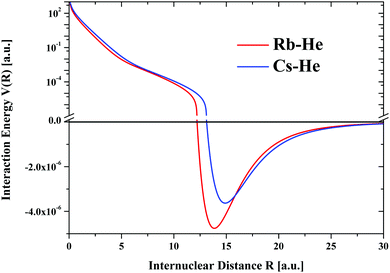Fig. 6 The interaction potentials of the ground state He–Rb and He–Cs predicted by the TTS potential model with Re, De, ZA, ZB listed in Table 4.
Table 7 Comparisons of the present predicted Re and De for RbHe and CsHe with the available data in the literatures49,72–76
RbHe CsHe
Re De × 106 Re De × 106
Present 13.86 4.76 14.89 3.63
Ayed et al.72 13.0 8.72 14.86 6.15
Pascale73 14.0 6.83 15.0 5.92
Baylis74 11.6 11.85 12.0 11.39
Kleinekathofer et al.49 14.24 3.71 15.02 3.17
Cvetko et al.75 13.86 4.92 14.61 4.14
Patil76 13.89 4.48 14.61 3.82

## VIII. Conclusion and discussion

In this paper we have given a historical account of our efforts to develop a potential model that is valid from R = 0 to R → ∞. In addition, we have given some new results of atom–atom interactions. We wish to point out that many developed formalisms are equally valid for ion–atom potentials.

In the introduction, we mentioned that the foundation of the theory of interatomic potential was laid in 1927 by Heitler and London in their study of H2 molecule using symmetrized wavefunction. In the following year, 1928, Pauling77 applied the Heitler–London theory to study the H2+ system. Although in this case, the approach is now commonly known as the linear combination of atomic orbitals, the formalism is the same. Thus, if the singlet state and triplet state wavefunctions of the H2 molecule are replaced by the gerade and ungerade state wavefunctions, respectively, of the H2+ molecular ion, all the formulae derived for H2 are equally applicable to H2+. The specific results are of course different.

The most obvious difference is that for ion–atom systems, in addition to dispersion attraction, they also have induction attraction. Other than that, most of the present formalisms are equally applicable to ion–atom systems. For example, the alkali ion–rare gas and halogen ion–rare gas systems were studied with the TT potential model by Ahlrichs et al..78 It will be interesting to study these systems with TTY potential since the Herring type exchange terms for ion–atom systems are also availabe.79,80 In fact, we have used the Generalized Heitler–London theory to study the alkali dimer cations and obtained very good results.81,82

## Conflicts of interest

There are no conflicts of interest to declare.

## Acknowledgements

X. W. S thanks the support of the Anhui Provincial Natural Science Foundation (No. 2008085MA25) and the National Natural Science Foundation of China (No. 11404006).

## References

1. R. R. Feynman, R. B. Leighton and M. Sands, The Feynman Lectures on Physics, Addison-Wesley, Reading, Mass, 1964, vol. I, ch. 1 Search PubMed.
2. D. M. Ceperley and H. Partridge, J. Chem. Phys., 1986, 84, 820 Search PubMed.
3. W. Cencek, M. Przybytek, J. Komasa, J. B. Mehl, B. Jeziorski and K. Szalewicz, J. Chem. Phys., 2012, 136, 224303 Search PubMed.
4. K. Patkowski and K. Szalewicz, J. Chem. Phys., 2010, 133, 094394 Search PubMed.
5. K. Patkowski, G. Murkachaew, C. M. Fou and K. Szalewicz, Mol. Phys., 2005, 103, 2031 Search PubMed.
6. R. Hellmann, B. Jäger and E. Bich, J. Chem. Phys., 2017, 147, 034304 Search PubMed.
7. L. Shirekov and V. Sladek, J. Chem. Phys., 2017, 147, 174103 Search PubMed.
8. W. Heitler and F. London, Z. Phys., 1927, 44, 455 Search PubMed.
9. S. C. Wang, Z. Phys., 1927, 28, 663 Search PubMed.
10. P. E. Siska, J. M. Parson, T. P. Schafer and Y. T. Lee, J. Chem. Phys., 1971, 55, 5672 Search PubMed.
11. J. M. Farrar and Y. T. Lee, J. Chem. Phys., 1972, 56, 5801 Search PubMed.
12. H. J. M. Hanley, J. A. Barker, J. M. Parson, Y. T. Lee and M. L. Klein, Mol. Phys., 1972, 24, 11 Search PubMed.
13. J. A. Barker, in Rare Gas Solids, ed. M. L. Klein and J. A. Vebables, Academic, New York, 1976, vol. 1, ch. 4 Search PubMed.
14. J. P. Toennies, Chem. Phys. Lett., 1973, 20, 238 Search PubMed.
15. F. London, Trans. Faraday Soc., 1937, 33, 8 Search PubMed.
16. H. Margenau, Rev. Mod. Phys., 1939, 11, 1 Search PubMed.
17. A. Dalgarno and J. T. Lewis, Proc. Phys. Soc., London, Sect. A, 1956, 67, 37 Search PubMed.
18. K. T. Tang and J. P. Toennies, J. Chem. Phys., 1978, 68, 5501 Search PubMed.
19. A. J. Thakkar, J. Chem. Phys., 1982, 89, 2092 Search PubMed.
20. S. H. Patil and K. T. Tang, J. Chem. Phys., 1997, 107, 3804 Search PubMed.
21. K. T. Tang and J. P. Toennies, J. Chem. Phys., 1977, 66, 1496 Search PubMed.
22. P. Habitz, K. T. Tang and J. P. Toennies, Chem. Phys. Lett., 1982, 85, 461 Search PubMed.
23. K. T. Tang and J. P. Toennies, J. Chem. Phys., 1981, 74, 1148 Search PubMed.
24. M. Faubel, K. H. Kohl, J. P. Toennies, K. T. Tang and Y. Y. Yung, Faraday Discuss. Chem. Soc., 1982, 73, 205 Search PubMed.
25. J. Hepburn, G. Scoles and R. Penco, Chem. Phys. Lett., 1975, 36, 451 Search PubMed.
26. R. Ahlrichs, R. Penco and G. Scoles, Chem. Phys., 1977, 19, 119 Search PubMed.
27. R. A. Aziz and C. C. Chen, J. Chem. Phys., 1977, 67, 5719 Search PubMed.
28. R. A. Aziz, in Rare gases; Potentials, Dynamics and Energy Transfer in Doped Crystals, ed. M. Klein, Springer, Berlin, 1984, p. 5 Search PubMed.
29. J. O. Hirschfelder, C. F. Curtiss and R. B. Bird, Molecular Theory of Gases and Liquids, Wily, New York, 1954. pp. 881–960 Search PubMed.
30. Y. S. Kim and R. G. Gordon, J. Chem. Phys., 1974, 61, 1 Search PubMed.
31. K. T. Tang and J. P. Toennies, J. Chem. Phys., 1984, 80, 3726 Search PubMed.
32. A. Koide, W. J. Meath and A. R. Allnatt, Chem. Phys., 1981, 58, 105 Search PubMed.
33. M. Gutkowski, J. Verbeek, J. H. Van Lenthe and G. Chałasinski, Mol. Phys., 1987, 111, 271 Search PubMed.
34. N. Jacobi and G. Csannak, Chem. Phys. Lett., 1975, 30, 367 Search PubMed.
35. K. T. Tang and J. P. Toennies, Z. Phys. D: At., Mol. Clusters, 1986, 1, 91 Search PubMed , There is a simple program in the appendix of this reference that converts Re and De into A and b.
36. K. T. Tang and J. P. Toennies, Mol. Phys., 2008, 106, 1645 Search PubMed.
37. X. W. Sheng, P. Li and K. T. Tang, J. Chem. Phys., 2009, 130, 174310 Search PubMed.
38. D. D. Yang, P. Li and K. T. Tang, J. Chem. Phys., 2009, 131, 154301 Search PubMed.
39. G. P. Yin, P. Li and K. T. Tang, J. Chem. Phys., 2010, 132, 074303 Search PubMed.
40. P. Li, W. Xie and K. T. Tang, J. Chem. Phys., 2010, 133, 084308 Search PubMed.
41. P. Li, J. Ren, N. Niu and K. T. Tang, J. Phys. Chem. A, 2011, 115, 6927 Search PubMed.
42. L. M. Wei, P. Li, L. W. Qiao and K. T. Tang, J. Chem. Phys., 2013, 139, 154306 Search PubMed.
43. K. T. Tang and J. P. Toennies, J. Chem. Phys., 1991, 95, 5918 Search PubMed.
44. K. T. Tang and J. P. Toennies, Chem. Phys. Lett., 1991, 175, 511 Search PubMed.
45. K. T. Tang, J. P. Toennies and C. L. Yiu, Int. Rev. Phys. Chem., 1998, 17, 363 Search PubMed.
46. K. T. Tang, J. P. Toennies and C. L. Yiu, J. Chem. Phys., 1993, 99, 377 Search PubMed.
47. D. R. Bates and A. Damgaard, Philos. Trans. R. Soc., A, 1949, 242, 101 Search PubMed.
48. E. L. Duman and B. M. Smirnov, Opt. Spectrosc., 1970, 29, 229 Search PubMed.
49. U. Kleinekathöfer, K. T. Tang, J. P. Toennies and C. L. Yiu, Chem. Phys. Lett., 1996, 249, 257 Search PubMed.
50. C. Herring and M. Flicker, Phys. Rev., 1964, 134, A362 Search PubMed.
51. C. Herring, Rev. Mod. Phys., 1962, 34, 631 Search PubMed.
52. C. Herring, in Magnetism, ed. G. Rado and H. Suhl, Academic, New York, 1966, vol. 2B, p. 1 Search PubMed.
53. W. Kolos and L. Wolniewicz, J. Chem. Phys., 1974, 43, 2427 (Chem. Phys. Lett., 1974, 24, 457) Search PubMed.
54. B. M. Smirnov and M. I. Chibisov, Sov. Phys. JETP, 1965, 21, 624 Search PubMed.
55. U. Kleinekathöfer, K. T. Tang, J. P. Toennies and C. L. Yiu, J. Chem. Phys., 1995, 103, 6617 Search PubMed.
56. U. Kleinekathöfer, K. T. Tang, J. P. Toennies and C. L. Yiu, J. Chem. Phys., 1997, 107, 9502 Search PubMed.
57. K. T. Tang, J. P. Toennies and C. L. Yiu, Phys. Rev. Lett., 1995, 74, 1546 Search PubMed.
58. J. B. Anderson, C. A. Traynor and B. M. Boghosian, J. Chem. Phys., 1993, 99, 345 Search PubMed.
59. Y. M. Chen, X. Y. Kuang, X. W. Sheng and X. Z. Yan, J. Phys. Chem. A, 2014, 118, 592 Search PubMed.
60. S. Warnecke, K. T. Tang and J. P. Toennies, J. Chem. Phys., 2015, 142, 131102 Search PubMed.
61. J. F. Peng, P. Li, J. Ren, L. W. Qiao and K. T. Tang, J. At. Mol. Sci., 2011, 2, 289 Search PubMed.
62. J. Goodisman, Diatomic Interaction Potential Theory, Academic Press, New York, 1973 Search PubMed.
63. R. A. Buckingham, Trans. Faraday Soc., 1958, 54, 453 Search PubMed.
64. X. W. Sheng, J. P. Toennies and K. T. Tang, Phys. Rev. Lett. Search PubMed , submitted.
65. N. Hishinuma, J. Chem. Phys., 1981, 75, 4960 Search PubMed.
66. J. P. Toennies, W. Welz and G. Wolf, J. Chem. Phys., 1979, 71, 614 Search PubMed.
67. K. T. Tang and J. P. Toennies, Chem. Phys., 1991, 156, 413 Search PubMed.
68. D. Das, A. F. Wagner and A. C. Wahl, J. Chem. Phys., 1978, 68, 4917 Search PubMed.
69. H. Partridge, J. R. Stallcop and E. Levin, J. Chem. Phys., 2001, 115, 6471 Search PubMed.
70. L. M. Wei, P. Li and K. T. Tang, Chem. Phys. Lett., 2017, 675, 40 Search PubMed.
71. J. Jiang, J. Mitroy, Y. Cheng and M. W. J. Bromley, At. Data Nucl. Data Tables, 2015, 101, 158 Search PubMed.
72. M. B. H. Ayed, R. Hamdi, H. Ghalla, B. Oujia and F. X. Gadeam, Theor. Chem. Acc., 2018, 137, 83 Search PubMed.
73. J. Pascale, Phys. Rev. A: At., Mol., Opt. Phys., 1983, 28, 632 Search PubMed.
74. W. E. Baylis, J. Chem. Phys., 1969, 51, 2665 Search PubMed.
75. D. Cveto, A. Lausi, A. Nirgabtem, F. Tommasini, P. Cortona and M. G. Dondi, J. Chem. Phys., 1994, 100, 2052 Search PubMed.
76. S. H. Patil, J. Chem. Phys., 1991, 94, 8089 Search PubMed.
77. L. Pauling, Chem. Rev., 1928, 5, 173 Search PubMed.
78. R. Ahlichs, H. J. Böhm, S. Bode, K. T. Tang and J. P. Toennies, J. Chem. Phys., 1988, 88, 6290 Search PubMed.
79. K. T. Tang, J. P. Toennies, M. Wanschura and C. L. Yiu, Phys. Rev. A: At., Mol., Opt. Phys., 1992, 46, 3746 Search PubMed.
80. T. C. Chang and K. T. Tang, J. Chem. Phys., 1995, 103, 10580 Search PubMed.
81. Ch Johann, U. Kleinekathöfer, K. T. Tang and J. P. Toennies, Chem. Phys. Lett., 1996, 257, 651 Search PubMed.
82. C. Johann, S. H. Patil, K. T. Tang and J. P. Toennies, Chem. Phys. Lett., 1998, 298, 158 Search PubMed.

### Footnote

This paper is written for the celebration of the 90th birthday of Prof. J. P. Toennies who has been our inspiration and mentor for almost half a century.
 This journal is © the Owner Societies 2020